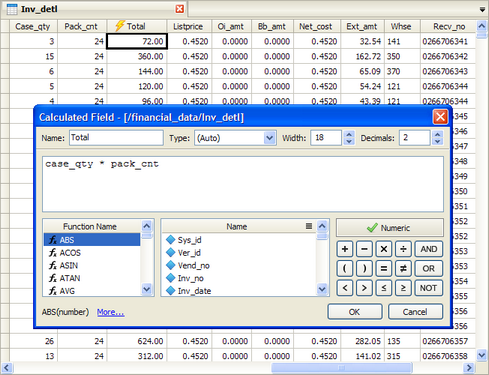﻿ Defining the Contents of a Calculated Field
 Defining the Contents of a Calculated Field

Performing Standard Data Operations > Creating Calculations > Defining the Contents of a Calculated Field

When you create a calculated field, a formula builder will open.  The formula builder is the dialog where you specify the actual formula, as well as other field definitions, such as field name and type.Field Name

When you create or edit a calculated field, you can change the field name using the "Name" text box at the top left of the dialog.  In addition to the dynamic nature of the calculation, the field name also changes in the table as you type.  In the example above, the calculated field name is "Total."

Field Type

As with standard fields, calculated fields also need to have a data type associated with them.  A data type is automatically generated depending on the formula that you use.  This automatic setting is signified by the word "Auto" in the "Type" drop-down box on the top of the dialog.  However, you may also change the type setting manually if you wish.  This is usually not necessary for normal use; however, it is important to have in certain circumstances, particularly when interacting with external database systems.  Click on the drop-down menu and select from the following types:

 • Character - This is a standard character type.
 • Wide Character - This is a character type, most often used with unicode characters.
 • Numeric - This is a standard numeric type, which will provide a precise decimal place.  For the number 1.234, if you choose 2 decimal places, the number will be saved as 1.23; the remaining decimal place will not be able to be retrieved again.
 • Double - This is a numeric type, which is more flexible.  For the number 1.234, if you choose 2 decimal places, the number will be shown as 1.23; however, if you change the decimal precision to 3, the number 1.234 will again be displayed.
 • Integer - This is a numeric type that has no decimal places.
 • Date - This is a date type that only shows the date values.
 • Datetime - This is date type that shows both date and time values.
 • Boolean - This is a logical true/false condition.

Field Width and Decimal Precision

The field width default is set to 30 characters for character and date types, 18 characters and 2 decimal places for numeric types, and 1 character for Boolean types.

However, you can edit these values to any number you wish to choose by clicking on the "Width" and "Decimal" text boxes and changing the value, either by manually typing in the new number or by using the arrows to increase or decrease the number.

Formula Box

The formula box is the place where you enter your formula.  You can type the formula in directly or you can also double-click on the helper items, such as the function names, field names and operators located underneath the formula box. Click here for further information on using formulas.

Formula Builder Help